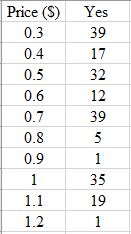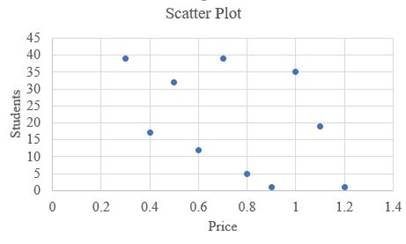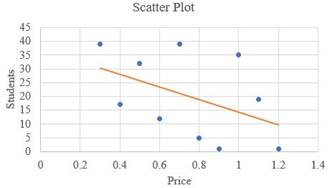# Make a table of the student who says “yes” at each price level.### Precalculus: Mathematics for Calcu...

6th Edition
Stewart + 5 others
Publisher: Cengage Learning
ISBN: 9780840068071### Precalculus: Mathematics for Calcu...

6th Edition
Stewart + 5 others
Publisher: Cengage Learning
ISBN: 9780840068071

#### Solutions

Chapter 1, Problem 13P

a.

To determine

## Make a table of the student who says “yes” at each price level.

Expert Solution

The table is,### Explanation of Solution

Given: The price table,

PriceYes or No30¢40¢50¢60¢70¢80¢90¢$1.0$1.1$1.2 Calculation: The function “RANDBETWEEN(1,40)” is used in the excel to make a table. Let’s take there are 200 students in the class. During the survey 39 students say “yes” to pay 30¢ , 17 students say “yes” to pay 40¢ , 32 students say “yes” to pay 50¢ , 12 students say “yes” to pay 60¢ , 39 students say “yes” to pay 70¢ , 5 students say “yes” to pay 80¢ , 1 students say “yes” to pay 90¢ , 35 students say “yes” to pay$1.0 , 19 students say “yes” to pay $1.1 , and 1 students say “yes” to pay$1.2 for a candy bar.

b.

To determine

### Sketch a scatter plot for a data which is found in part (a).

Expert Solution

The graph is,### Explanation of Solution

Given: The data which is found in part (a),

PriceYes30¢3640¢1050¢660¢1070¢1580¢1290¢38$1.03$1.124$1.27 Calculation: Using Excel to scetch a scatter plot. • Write the data in the excel and plot using chart “scatter”. c. To determine ### Draw a regression line. Expert Solution ## Answer to Problem 13P The solution is,### Explanation of Solution Given: The data set is, PriceYes30¢3640¢1050¢660¢1070¢1580¢1290¢38$1.03$1.124$1.27

Calculation:

The regression line is defined as,

y'=a+bt     .....(1)

Find the value of a and b using relation,

a=(y)(x2)(x)(xy)n(x2)(x)2b=n(xy)(x)(y)n(x2)(x)2

After simplifying the value of a and b, put into the equation (1) and get the solution.

Using Excel to scetch a scatter plot.

d.

To determine

### Explain what is p-intercept of the demand equation.

Expert Solution

The price-intercept is defined as the price at which quantity demand equals to zero.

### Explanation of Solution

Given: The data set is,

PriceYes30¢3640¢1050¢660¢1070¢1580¢1290¢38$1.03$1.124$1.27 Calculation: The quantity demand equation is defined as, Qd=abP Where, Qd is the quantity demand, a is the maximum value, b is the slope of the data set. P is the price-intercept. Let’s take Qd=0 to calculate price-intercept then, 0=38(12.1212)PP=38012.1212P=3.135$

### Have a homework question?

Subscribe to bartleby learn! Ask subject matter experts 30 homework questions each month. Plus, you’ll have access to millions of step-by-step textbook answers!